Assignment 5: Classifications
Share
Explore#PROBLEM 2: Bank MarketingYu Zhu
|
This dataset captures the results of a series of direct marketing campaigns of “BANK”, an international banking institution. The campaigns were based on phone calls. Often, more than one contact to the same client was required, in order to assess if the product (bank term deposit) would be ('yes') or not ('no') subscribed.
Run the a classifier that predicts the subscription of a term deposit using a 90:10, 80:20, and 70:30 split strategy
#preparations -------------------------------------------------------------------------
library(tidyverse)
library(fastDummies)
library(visdat)
library(caret) #confusion matrix package
library(rpart.plot)
library(rpart)

dfbank[dfbank==""]=NA
vis_miss(dfbank,warn_large_data=FALSE) #no NA value
summary(dfbank)

#90-10---------------------------------------------------------------------------------
set.seed(97) # setting seed to reproduce results of random sampling
split=(.9) #90/10
trainingRowIndex = sample(1:nrow(dfbank),(split)*nrow(dfbank)) # row indices for training data
trainingData = dfbank[trainingRowIndex, ] # model training data
testData = dfbank[-trainingRowIndex, ] # test data

dtree = rpart(y ~age+job+marital+default+housing+loan+contact+duration+pdays+previous+poutcome+nr.employed, data=trainingData, method="class",parms=list(split="information"), control=rpart.control(minsplit=2, cp=0.001))
plotcp(dtree) # when cp=0.006 the model performs best
dtree\$cptable
dtree\$variable.importance # the importance of variables in this model is duration>nr.employed>pdays
dtree.pruned = prune(dtree, cp=.006) # pruning the decision tree

prp(dtree.pruned, type = 2, extra = 104,fallen.leaves = TRUE, main="Decision Tree") #plot the decision tree
dtree.pred = predict(dtree.pruned, testData, type="class") #predict based on model

conMatrix = confusionMatrix(data=dtree.pred, reference = testData\$y) #Confusion Matrix
print(conMatrix)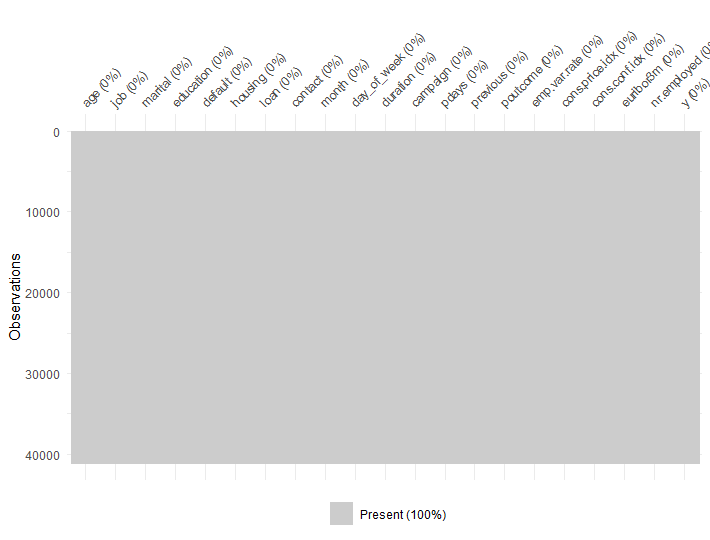This plot shows no NA value in this dataset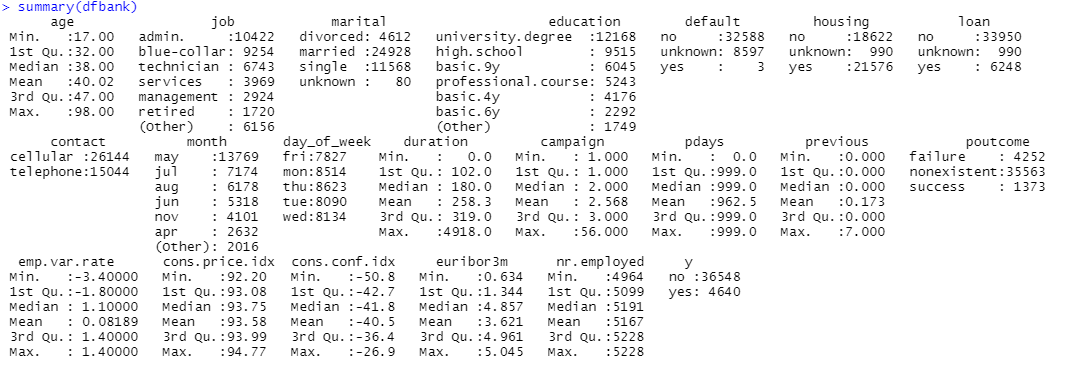The summary result shows the basic situations about this dataset, we can find the result of subscription is not balanced, about 90% is no and only 10% is yes, so we can not say it’s a good model if the accuracy is near 90%, because even if I randomly guess all result is no, the prediction accuracy is still about 90%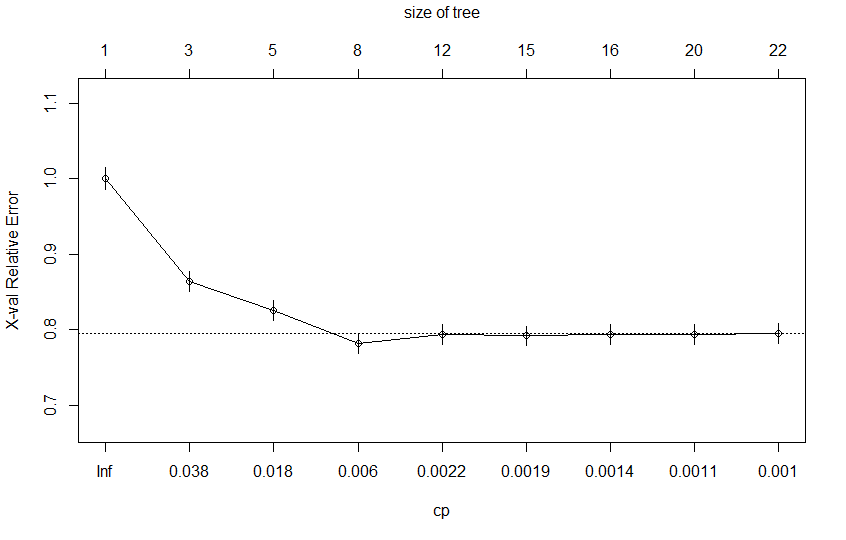This plot shows when cp=0.006 the model performs best, therefore when we prune the decision tree model, we can use cp = 0.006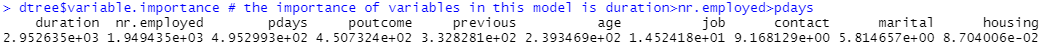The result shows the importance of variables in this model is duration>nr.employed>pdays>........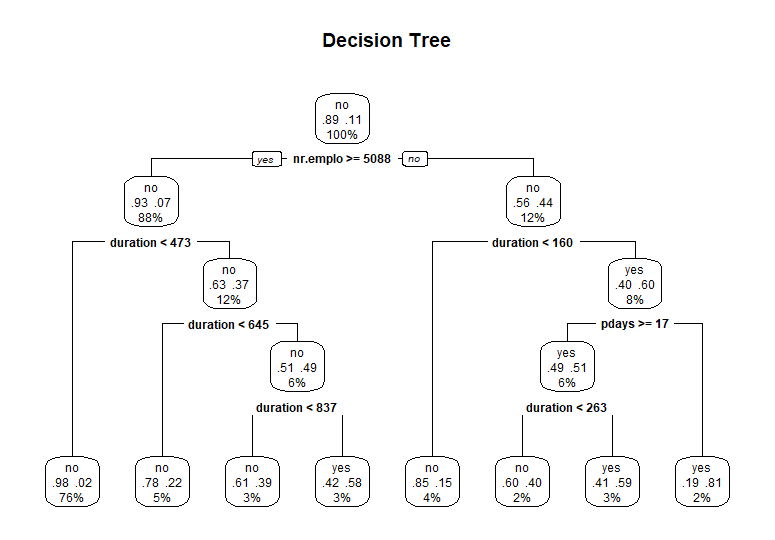This is the decision tree plot after the pruning.
From this plot, we notice that the tree first split the data by nr.emplo then by duration and pdays.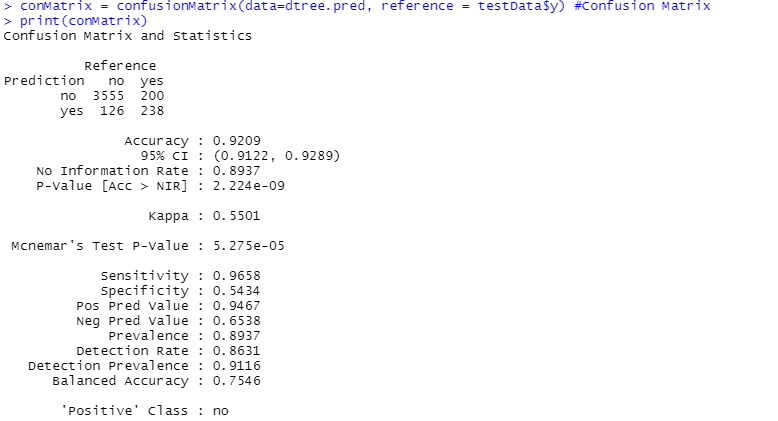This is the Confusion Matrix of prediction result on testing data, we can find the accuracy is 92.09%
Precision Rate = TP/(TP+FP)= 65.38%
Recall Rate= TP/(TP+FN)= 54.33%
From the result we can see that it’s very likely for this model to predict a NO result when it is a YES in real.

#80/20---------------------------------------------------------------------------------
set.seed(97)
split=(.8) #80/20
trainingRowIndex = sample(1:nrow(dfbank),(split)*nrow(dfbank)) # row indices for training data
trainingData = dfbank[trainingRowIndex, ] # model training data
testData = dfbank[-trainingRowIndex, ] # test data

dtree = rpart(y ~age+job+marital+default+housing+loan+contact+duration+pdays+previous+poutcome+nr.employed, data=trainingData, method="class",parms=list(split="information"), control=rpart.control(minsplit=2, cp=0.001))
plotcp(dtree) # when cp=0.0069 the model performs best
dtree\$cptable
dtree\$variable.importance # the importance of variables in this model is duration>nr.employed>pdays
dtree.pruned = prune(dtree, cp=0.0069) # pruning the decision tree

prp(dtree.pruned, type = 2, extra = 104,fallen.leaves = TRUE, main="Decision Tree") #plot the decision tree
dtree.pred = predict(dtree.pruned, testData, type="class") #predict based on model

conMatrix = confusionMatrix(data=dtree.pred, reference = testData\$y) #Confusion Matrix
print(conMatrix)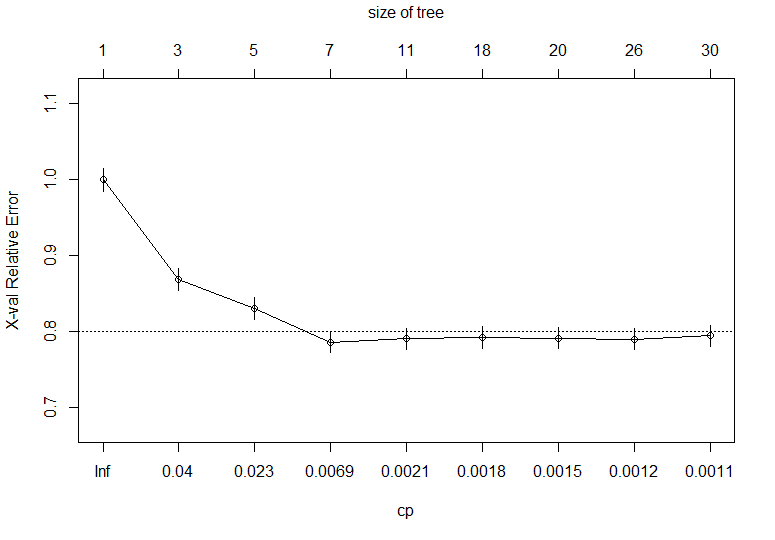This plot shows when cp=0.0069 the model performs best, therefore when we prune the decision tree model, we can use cp = 0.0069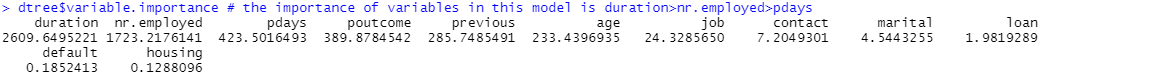The result shows the importance of variables in this model is duration>nr.employed>pdays>........, in another word, duration influence the result mostly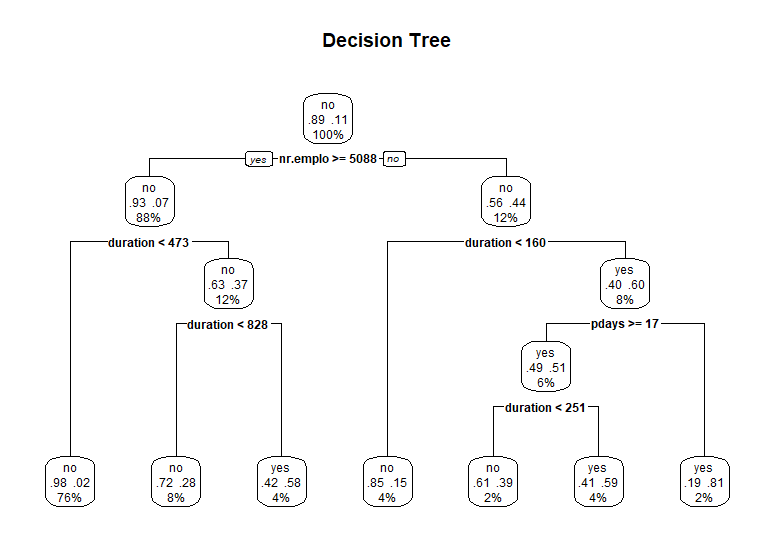This is the decision tree plot after the pruning.
From this plot, we notice that the tree first split the data by nr.emplo then by different section of duration and pdays.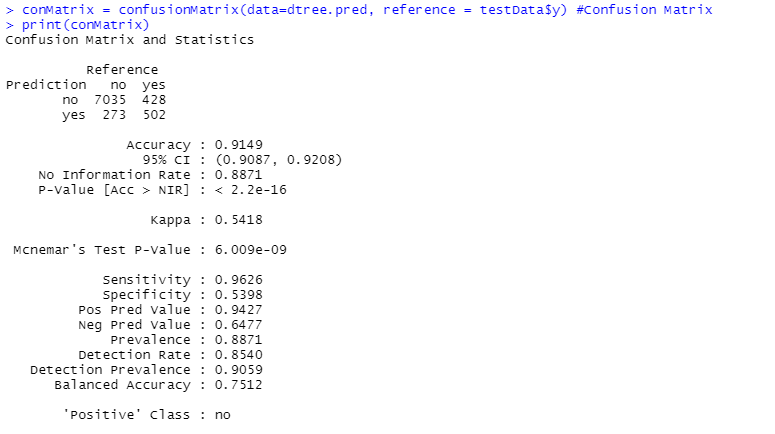This is the Confusion Matrix of prediction result on testing data, we can find the accuracy is 91.49%
Precision Rate = TP/(TP+FP)= 64.77%
Recall Rate= TP/(TP+FN)= 53.98%
From the result we can see that it’s very likely for this model to predict a NO result when it is a YES in real.

#70/30---------------------------------------------------------------------------------
set.seed(97)
split=(.7) #70/30
trainingRowIndex = sample(1:nrow(dfbank),(split)*nrow(dfbank)) # row indices for training data
trainingData = dfbank[trainingRowIndex, ] # model training data
testData = dfbank[-trainingRowIndex, ] # test data

dtree = rpart(y ~age+job+marital+default+housing+loan+contact+duration+pdays+previous+poutcome+nr.employed, data=trainingData, method="class",parms=list(split="information"), control=rpart.control(minsplit=2, cp=0.001))
plotcp(dtree) # when cp=0.01 the model performs best
dtree\$cptable
dtree\$variable.importance # the importance of variables in this model is duration>nr.employed>pdays
dtree.pruned = prune(dtree, cp=0.01) # pruning the decision tree

prp(dtree.pruned, type = 2, extra = 104,fallen.leaves = TRUE, main="Decision Tree") #plot the decision tree
dtree.pred = predict(dtree.pruned, testData, type="class") #predict based on model

conMatrix = confusionMatrix(data=dtree.pred, reference = testData\$y) #Confusion Matrix
print(conMatrix)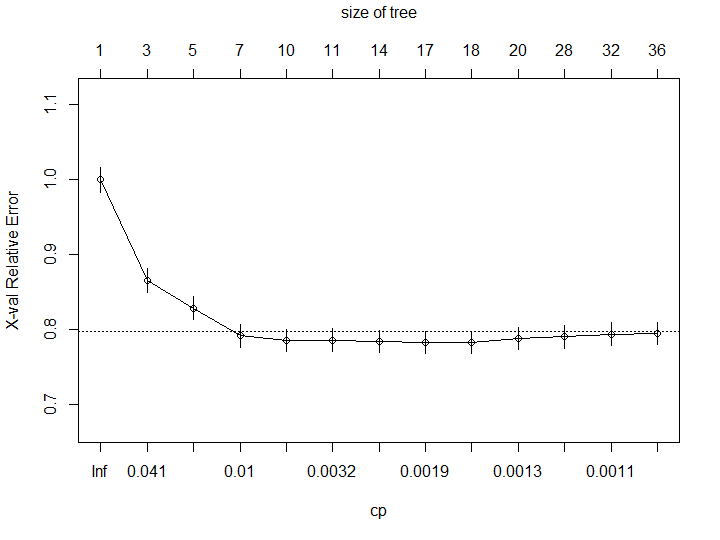This plot shows when cp=0.01 the model performs best, therefore when we prune the decision tree model, we can use cp = 0.01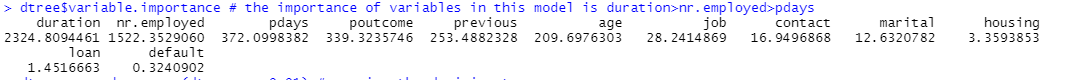The result shows the importance of variables in this model is duration>nr.employed>pdays>........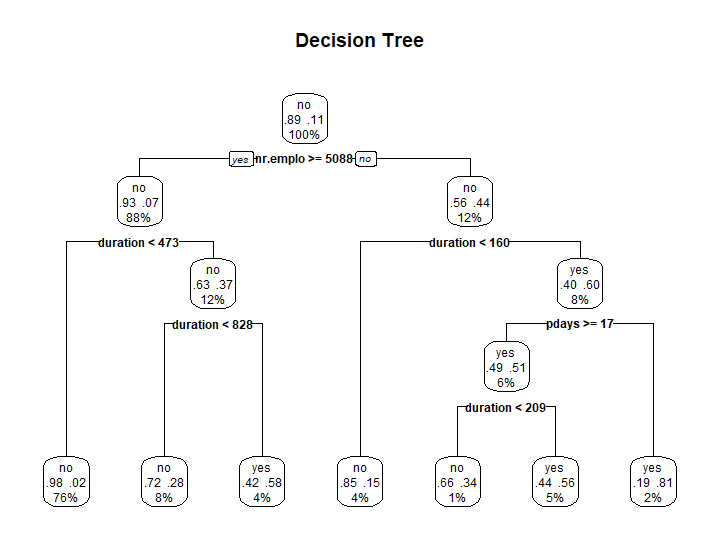This is the decision tree plot after the pruning.
From this plot, we notice that the tree first split the data by nr.emplo then by different section of duration and pdays.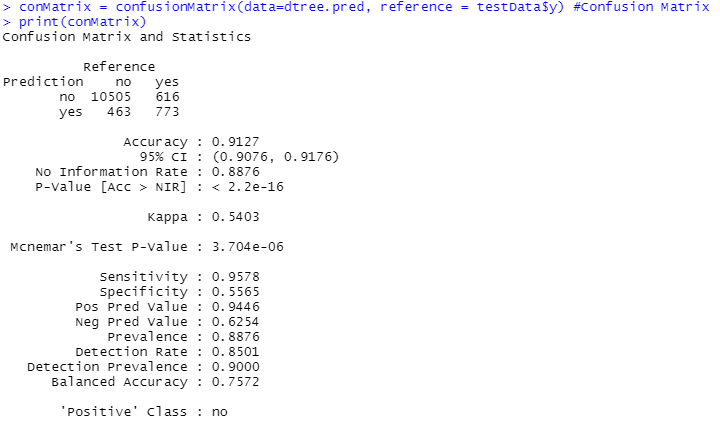This is the Confusion Matrix of prediction result on testing data, we can find the accuracy is 91.27%
Precision Rate = TP/(TP+FP)= 62.54%
Recall Rate= TP/(TP+FN)= 55.65%
From the result we can see that it’s very likely for this model to predict a NO result when it is a YES in real.

2. Interpret the classification trees and describe their accuracy.